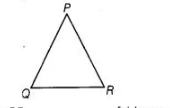# In ΔPQR, if ∠R > ∠Q, then`
Question:

In ΔPQR, if ∠R > ∠Q, then

(a) QR > PR

(b) PQ > PR

(c) PQ < PR

(d) QR < PR

Solution:

(b) Given, $\angle R>\angle Q$$\Rightarrow \quad P Q>P R \quad$ [side opposite to greater angle is longer]